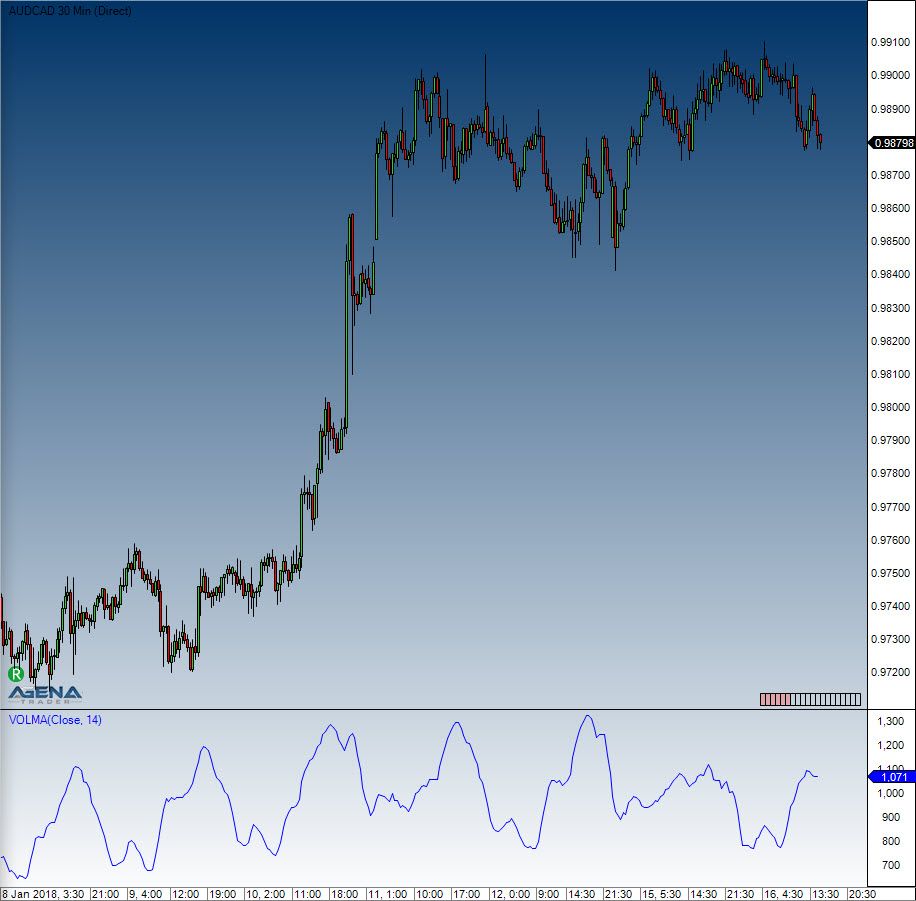Comment on page

# Volume Moving Average (VOLMA)

#### Description

The VOLMA calculation is carried out by applying an exponential moving average to the respective volume of each period, that is to say its EMA (volume).
Caution: In trading literature, the Volume Moving Average is often confused with the abbreviation for the Variable Moving Average (VMA).

#### Interpretation

The VOLMA indicator helps you to find and assess the relative volume of a period.
Some simple guidelines should be followed:
The volume should be above the VOLMA at a break
After a volume spike (high), an exhaustion phase will kick in. The exhaustion can lead to a complete reversal of the price movement. Generally speaking, however, this is simply a resting phase
After three successive volume spikes, it becomes much less likely that the situation will continue in the same direction
Here, you can find more general information about Moving Averages.

#### Further information

Volumen-Analyse.de: http://www.volumen-analyse.de

#### Usage

VOLMA(int period)
VOLMA(IDataSeries inSeries, int period)
VOLMA(int period)[int barsAgo]
VOLMA(IDataSeries inSeries, int period)[int barsAgo]

#### Return value

double
When using this method with an index (e.g. VOLMA(14)[int barsAgo] ), the value of the indicator will be issued for the referenced bar.

#### Parameters

inSeries Input data series for the indicator
period Number of bars included in the calculations

#### VisualizationVolume Moving Average (VOLMA)

#### Example

//Output for the value of the Volume Moving Average (VOLMA)
Print("The current VOLMA value is " + VOLMA(14));